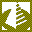Homepage » Education » Scientific Calculator - ScienCalc 1.3.6ScienCalc is a convenient and powerful scientific calculator.

### Scientific Calculator - ScienCalc OverviewScientific Calculator - ScienCalc 1.3.6 was downloaded & scanned by our team, against different types of malwares (like viruses, spyware, trojans, backdoors and others) and found CLEAN.

ScienCalc is a convenient and powerful scientific calculator. ScienCalc calculates mathematical expression. It supports the common arithmetic operations (+, -, *, /) and parentheses.

The program contains high-performance arithmetic, trigonometric, hyperbolic and transcendental calculation routines. All the function routines therein map directly to Intel 80387 FPU floating-point machine instructions.

Find values for your equations in seconds:
Scientific calculator gives students, teachers, scientists and engineers the power to find values for even the most complex equation set. You can build equation set, which can include a wide array of linear and nonlinear models for any application:

*Linear equations
*Polynomial and rational equations
*Nonlinear exponential, logarithmic and power equations
*Pre-defined mathematical an ...

 Publisher Website: Institute of Mathematics and Statistics Category: Education License: shareware (\$15) Operating Systems: Win2000, Win7 x32, Win98, WinOther, WinVista, WinXP, Other Filename: calculator.exe Size: 2.54 MB MD5 Checksum: a5227267ea1db2d57ae243424a2ea2ac Last Updated: 05 Apr 2011 Screenshot: Scientific Calculator - ScienCalc 1.3.6 Screenshot

Full Description: ScienCalc is a convenient and powerful scientific calculator. ScienCalc calculates mathematical expression. It supports the common arithmetic operations (+, -, *, /) and parentheses.

The program contains high-performance arithmetic, trigonometric, hyperbolic and transcendental calculation routines. All the function routines therein map directly to Intel 80387 FPU floating-point machine instructions.

Find values for your equations in seconds:
Scientific calculator gives students, teachers, scientists and engineers the power to find values for even the most complex equation set. You can build equation set, which can include a wide array of linear and nonlinear models for any application:

*Linear equations
*Polynomial and rational equations
*Nonlinear exponential, logarithmic and power equations
*Pre-defined mathematical and physical constants

Understandable and convenient interface:
A flexible work area lets you type in your equations directly. It is as simple as a regular text editor. Annotate, edit and repeat your calculations in the work area. You can also paste your equations into the editor panel.
Example of mathematical expression:
5.44E-4 * (x - 187) + (2 * x) + square(x) + sin(x/deg) + logbaseN(6;2.77)
History of all calculations done during a session can be viewed. Print your work for later use. Comprehensive online help is easily accessed within the program.

Features:
--------
*Scientific calculations
*Unlimited expression length
*Parenthesis compatible
*Scientific notation
*More than 35 functions
*More than 40 constants
*User-friendly error messages
*Simple mode (medium size on desktop)
*Paste expressions into ScienCalc
*Comprehensive documentation
*All the benefits that Windows bestows, such as multi-tasking and print formatting are available

### Changelog

Version: 1.3.6 (21 Mar 2011)
Version: 1.3.4 (26 Jan 2010)
Version: 1.3.3 (27 Jul 2009)
Version: 1.3

### Why to stay up-to-date?

Most of the time having the latest version of a software is a good thing. Usually software updates add new features, which makes the software more usefull. Also with every update a software becomes more secure and more stable by fixing bugs & vulnerabilities from previous versions.

We offer you 3 options in order to stay up-to-date with your software list:

1. Using Update Notifier (recommended) - AUTOMATIC PROCESS
The Update Notifier is a FREE small utility that scans your computer for installed software and displays a list of updates.

2. Register on the website and use the Watch List Option - MANUAL PROCESS
Signup on the website for free and use Watch List option (a collection of software, made by you, where you can see the current version or download it.

3. By Email - SEMI-AUTOMATIC PROCESS
Complete the following form and we'll send you an email, every time a new version of Scientific Calculator - ScienCalc is launched.

### How can you help?

Because this is a free service and we intent to keep it free, we need your input. You can: